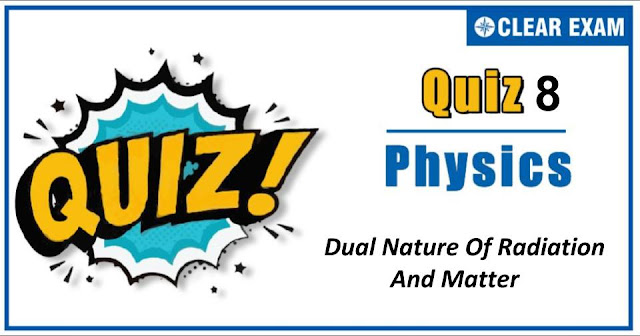## [LATEST]$type=sticky$show=home$rm=0$va=0$count=4$va=0

As per analysis for previous years, it has been observed that students preparing for NEET find Physics out of all the sections to be complex to handle and the majority of them are not able to comprehend the reason behind it. This problem arises especially because these aspirants appearing for the examination are more inclined to have a keen interest in Biology due to their medical background.

Furthermore, sections such as Physics are dominantly based on theories, laws, numerical in comparison to a section of Biology which is more of fact-based, life sciences, and includes substantial explanations. By using the table given below, you easily and directly access to the topics and respective links of MCQs. Moreover, to make learning smooth and efficient, all the questions come with their supportive solutions to make utilization of time even more productive. Students will be covered for all their studies as the topics are available from basics to even the most advanced.

Q71. Hard X-rays for the study of factures in bones should have a minimum wavelength of 10-11 m. The accelerating voltage for electrons in X-ray machine should be
•  <124.2 kV
•  >124.2 kV
•  Between 60 kV and 70 kV
•  =100 kV
Q72.e/m ratio of anode rays produced in a discharge tube, depends on the
•  Nature of the gas filled in the tube
•  Nature of the material of anode
•  Nature of the material of cathode
•  All the above
Solution
e/m of the anode rays depend on the nature of the gas filled in the discharge tube

Q73.  The continuous X-rays spectrum produced by an X-ray machine at constant voltage has
•   A maximum wavelength
•  A minimum wavelength
•  A single wavelength
•  A minimum frequency
Solution
Continuous spectrum of X-rays consists of radiations of all possible wavelength range having a definite short wavelength limit

Q74. The values +1/2 and -1/2 of spin quantum number show
•  Rotation of e- clockwise and anticlockwise direction respectively
•  Rotation of e- anticlockwise and clockwise directions respectively
•  Rotation in any direction according to convention
•  None of the above
Solution
Rotation in any direction according to convention

Q75.The de-Broglie wavelength associated with the particle of mass m moving with velocity v is
•  h/mv
•  mv/h
•  mh/v
•  m/hv
Solution
λ=h/p=h/mv

Q76. The cathode rays have particle nature because of the fact that
•  They can propagate in vacuum
•  They produced fluorescence
• C
Solution
They are deflected by electric and magnetic fields

Q77.A potential difference of 104 V is applied across an X-ray tube. The ratio of the de-Broglie wavelength of X-rays produced is (e/m for electron=1.8×1011 Ckg-1
•  -4V
•  -3 V
•  -2 V
•  -1 V
Solution
Stopping potential is that negative potential for which photo electric current is zero

Q78.Work function of a metal is 2.51 eV. Its threshold frequency
•  5.9×1014 cycles/s
•  6.5×1014 cycles/s
•  9.4×1014 cycles/s
•  6.08×1014 cycles/s
Q79.If gE and gM are the acceleration due to gravity on the surfaces of the earth and the moon respectively and it Millikan’s oil drop experiment could be performed on the two surfaces, one will find the ratio (electronic charge on the moon )/(electronic charge on the earth ) to be
•  1
•  Zero
•  gE/gM
•  gM/gE
Q80. λep and λα are the de Broglie wavelengths of electron, proton and α particle. If all are accelerated by same potential, then
•  λepα
•  λepα
• λepα
•  λepα
Solution
-->#### Written by: AUTHORNAME

AUTHORDESCRIPTION## Want to know more

Please fill in the details below:

## Latest IITJEE Articles$type=three$c=3$author=hide$comment=hide$rm=hide$date=hide$snippet=hide ## Latest NEET Articles$type=three$c=3$author=hide$comment=hide$rm=hide$date=hide$snippet=hide

Name

ltr
item
BEST NEET COACHING CENTER | BEST IIT JEE COACHING INSTITUTE | BEST NEET, IIT JEE COACHING INSTITUTE: Dual-Nature-Of-Radiation-and-Matter-Quiz-8